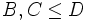# Modularity satisfies intermediate subgroup condition

This article gives the statement, and possibly proof, of a subgroup property (i.e., modular subgroup) satisfying a subgroup metaproperty (i.e., intermediate subgroup condition)
View all subgroup metaproperty satisfactions | View all subgroup metaproperty dissatisfactions |Get help on looking up metaproperty (dis)satisfactions for subgroup properties
Get more facts about modular subgroup |Get facts that use property satisfaction of modular subgroup | Get facts that use property satisfaction of modular subgroup|Get more facts about intermediate subgroup condition

## Statement

### Verbal statement

A modular subgroup of a group is also a modular subgroup inside every intermediate subgroup.

## Definitions used

### Modular subgroup

Further information: Modular subgroup

A subgroup$A$ of a group$G$ is termed modular in$G$ if for any subgroups$B, C \le G$ such that$A \le C$, we have:$\langle A, B \cap C \rangle = \langle A,B \rangle \cap C$.

### Intermediate subgroup condition

Further information: Intermediate subgroup condition

A subgroup property$p$ is said to satisfy the intermediate subgroup condition if whenever$A \le D \le G$ are groups such that$A$ satisfies property$p$ in$G$,$A$ also satisfies property$p$ in$D$.

## Proof

Given: A group$G$, subgroups$A \le D \le G$.$A$ is modular in$G$.

To prove:$A$ is modular in$D$: whenever$B, C \le D$ are such that$A \le C$, we have:$\langle A, B \cap C \rangle = \langle A, B \rangle \cap C$.

Proof: Since$B,C$ are subgroups of$D$, they are also subgroups of$G$, and the condition$A \le C$ holds by assumption. Thus, by modularity of$A$ in$G$, we conclude that:$\langle A, B \cap C \rangle = \langle A, B \rangle \cap C$.